• 2021-04-20 05:05:55

《matlab多元非线性回归教程》由会员分享，可在线阅读，更多相关《matlab多元非线性回归教程(2页珍藏版)》请在人人文库网上搜索。

1、matlab回归(多元拟合)教程前言 1、学三条命令 polyfit(x,y,n)-拟合成一元幂函数(一元多次) regress(y,x)-可以多元， nlinfit(x,y,fun,beta0) (可用于任何类型的函数，任意多元函数，应用范围最主，最万能的) 2、同一个问题，这三条命令都可以使用，但结果肯定是不同的，因为拟合的近似结果，没有唯一的标准的答案。相当于咨询多个专家。 3、回归的操作步骤： 根据图形(实际点)，选配一条恰当的函数形式(类型)-需要数学理论与基础和经验。(并写出该函数表达式的一般形式，含待定系数)-选用某条回归命令求出所有的待定系数。所以可以说，回归就是求待定系数的过。

2、程(需确定函数的形式) 一、回归命令 一元多次拟合polyfit(x,y,n);一元回归polyfit;多元回归regress-nlinfit(非线性) 二、多元回归分析 对于多元线性回归模型(其实可以是非线性，它通用性极高)： exxypp?110 设变量12,pxxx y的n组观测值为12(,)1,2,iiipixxxyin ? 记 ?npnnppxxxxxxxxxx?212222111211111，?nyyyy?21，则?p?10 的估计值为排列方式与线性代数中的线性方程组相同()，拟合成多元函数-regress 使用格式：左边用b=b, bint, r, rint, stats右边用=。

3、regress(y, x)或regress(y, x, alpha) -命令中是先y后x, -须构造好矩阵x(x中的每列与目标函数的一项对应) -并且x要在最前面额外添加全1列/对应于常数项 -y必须是列向量 -结果是从常数项开始-与polyfit的不同。) 其中： b为回归系数，?的估计值(第一个为常数项)，bint为回归系数的区间估计，r: 残差 ，rint: 残差的置信区间，stats: 用于检验回归模型的统计量，有四个数值：相关系数r2、F值、与F对应的概率p和残差的方差(前两个越大越好，后两个越小越好)，alpha: 显著性水平(缺省时为0.05，即置信水平为95%)，(alpha不。

4、影响b,只影响bint(区间估计)。它越小，即置信度越高，则bint范围越大。显著水平越高，则区间就越小)(返回五个结果)-如有n个自变量-有误(n个待定系数)，则b 中就有n+1个系数(含常数项，-第一项为常数项)(b-b的范围/置信区间-残差r-r的置信区间rint-点估计-区间估计 此段上课时不要：- 如果i?的置信区间(bint的第1i?行)不包含0，则在显著水平为?时拒绝0i?的假设，认为变量ix是显著的*(而rint残差的区间应包含0则更好)。b,y等均为列向量,x为矩阵(表示了一组实际的数据)必须在x第一列添加一个全1列。-对应于常数项-而nlinfit不能额外添加全1列。结果的系数就是与此矩阵相对应的(常数项，x1,x2,xn)。(结果与参数个数：1/5=2/3-y,x顺序-x要额外添加全1列) 而nlinfit:1/3=4-x,y顺序-x不能额外添加全1列，-需编程序，用于模仿需拟合的函数的任意形式，一定两个参数，一为系数数组，二为自变量矩阵(每列为一个自变量) 有n个变量-不准确，x中就有n列,再添加一个全1列(相当于常数项)，就变为n+1列，则结果中就有n+1个系数。 x需要经过加工，如添加全1列，可能还要添加其他需要的变换数据。 相关系数r2越接近1，说明回归方程越显著；(r2越大越接近1越好)F越大，说明回归。

更多相关内容
• 多变量线性回归，基于吴恩达的课程整理。使用个例子。
• 非线性回归问题（单变量多变量）可以使用图形用户界面 (GUI) 轻松提出，该界面使用以下求解器之一解决问题： - nlinfit：仅单变量问题。 - lsqnonlin：可以处理多变量问题（个因拟合变量，ydata为矩阵）。 - ...matlab
• 在#机器学习系列一#中，我们讨论了单变量线性回归，而多变量线性回归与单变量类似。首先我们来看一个例子，下表是波特兰的房子价格，针对不同的房屋面积和卧室间数。 房屋面积/ft2ft^2 卧室/间 价格/美元...

## 一、多变量的线性回归

在#机器学习系列一#中，我们讨论了单变量的线性回归，而多变量的线性回归与单变量类似，一致内容就不再赘述了。首先我们来看一个例子，下表是波特兰的房子价格，针对不同的房屋面积和卧室间数。

房屋面积/ ft2 $ft^2$卧室/间价格/美元房屋面积/ ft2 $ft^2$卧室/间价格/美元
2104339990019624259900
1600332990038903573900
2400336900011003249900
1416223200014583464500
3000453990025263469000
1985429990022003475000
1534331490026373299900
1427319899918392349900
1380321200010001169900
1494324250020404314900
1940423999931373579900
2000334700018114285900
1890332999914373249900
4478569990012393229900
1268325990021324345000
2300444990042154549000
1320229990021624287000
1236319990016642368500
2609449999822383329900
3031459900025674314000
1767325290012003299000
188822550008522179900
1604324290018524299900
12033239500

在这里我们先规定一下符号记法：

符号含义
m $m$ 训练样本的个数
n$n$每个训练样本的特征个数
x $x$ 训练样本中的输入变量
y$y$训练样本中的输出变量
(x(i),y(i)) $(x^{(i)},y^{(i)})$ i $i$个训练样本
x(i)j$x^{(i)}_j$ i $i$个训练样本的输入变量的第j$j$个特征
hθ(x) $h_\theta(x)$输入变量 x $x$与输出变量y$y$的映射关系

在本例中，训练样本个数 m $m$为47；输入变量的特征数n$n$为2，分别为房屋面积和卧室间数； x(i)1 $x^{(i)}_1$为第 i $i$个样本的房屋面积，x(i)2$x^{(i)}_2$为第 i $i$个样本的卧室间数，y(i)$y^{(i)}$为第 i $i$个样本的房屋价格，例x(1)=T$x^{(1)}=\begin{bmatrix}2104&3\end{bmatrix} ^ \mathrm{ T }$ y(1)=399900 $y^{(1)}=399900$。有了训练样本，下一步我们需要用一种算法得到一个比较好的映射关系 hθ(x) $h_\theta(x)$，当给定房屋面积和卧室间数 x $x^*$时，通过映射关系可以得到一个符合训练样本规律的房屋价格 y $y^*$。由于我们现在讨论的是多变量的线性回归，因此我们假设

hθ(x)=θ0+θ1x1+θ2x2(1)

同单变量线性回归一样，我们定义一个代价函数 J(θ0,θ1,θ2) $J(\theta_0,\theta_1,\theta_2)$。这样，问题就转化为寻找一组 θ0 $\theta_0$ θ1 $\theta_1$ θ2 $\theta_2$使得 J(θ0,θ1,θ2) $J(\theta_0,\theta_1,\theta_2)$最小。
J(θ0,θ1,θ2)=12mi=1m(hθ(x(i))y(i))2(2)
应用梯度下降法（ 详见机器学习系列一
θ0=θ0αθ0J(θ0,θ1)θ1=θ1αθ1J(θ0,θ1)(3)

经过严格推导可得
θ0=θ0αmi=1m(hθ(x(i))y(i))θ1=θ1αmi=1m(hθ(x(i))y(i))x(i)1θ2=θ2αmi=1m(hθ(x(i))y(i))x(i)2(4)

## 二、批处理

为了可以用矩阵进行批处理，我们把 x $x$增加一个维度x0=1$x_0=1$，这样

hθ(x)=θ0x0+θ1x1+θ2x2=[θ0θ1θ2]x0x1x2=θTx(5)

因此式（4）可以写成如下形式
θj=θjαmi=1m(hθ(x(i))y(i))x(i)j(6)

这样的话，输入变量 X $X$可以写成如下m×3$m\times3$矩阵形式（7），其中第一列为1，第二列为第一个特征（房屋面积），第三列为第二个特征（卧室间数）；输出变量 y $y$可以写成m×1$m\times1$矩阵；所求 θ $\theta$可以写成 3×1 $3\times1$矩阵，即 θ=[θ0θ1θ2]T $\theta=\begin{bmatrix}\theta_0&\theta_1&\theta_2\end{bmatrix} ^ \mathrm{ T }$
x(1)Tx(2)Tx(3)Tx(m)T(7)

此时式(2),(6)用matlab编程语言如下，非常简洁明了：

J = 1/(2*m)*(X*theta-y)'*(X*theta-y);
theta = theta-alpha/m*(X'*(X*theta-y));

## 三、特征缩放

由于这是多变量线性回归，还有一个比较棘手的问题，即不同的特征取值范围不一样。在本例中特征1取值范围0~3000，而特征2取值范围0~5，差距悬殊。在处理数据前若没有做特征缩放也就是没有做过标准化，目标函数则无法适当的运作。举例来说，利用两点间的距离计算两点的差异对数据进行分类，若其中一个特征具有非常广的范围，那两点间的差异就会被该特征左右，因此，所有的特征都该被标准化，这样才能大略的使各特征依比例影响距离。在本例中若不进行特征缩放，将会耗费很长很长的时间找到最优解，极端情况下甚至因为各种特征差距太大甚至无法找到。不单单是线性回归，很多情况下我们都要对数据进行预处理即特征缩放，可以说应用范围非常广。
常见的归一化方法有两种:
1. min-max标准化
min-max标准化也称为离差标准化，是利用样本数据的最大值和最小值对原始数据的线性变换。经过处理使结果值映射到[0 - 1]之间，转换函数如下：

x=xminmaxmin(8)

2. Z-score标准化
Z-score标准化利用原始数据的均值和标准差进行数据的标准化。经过处理的数据符合标准正态分布，即均值为0，标准差为1，转化函数为如下：
x=xμσ(9)

本例中，我们采用Z-score标准化，以第一个特征(房屋面积)为例
x1=x1μx1σx1=x1μx1σx1=x12000.68786.2

经过标准化，梯度下降法迭代，我们得到
θ=[8.9598×104139.21078.7380×103]T $\theta=\begin{bmatrix}8.9598\times10^4&139.2107&-8.7380\times10^3\end{bmatrix}^\mathrm{ T }$
当房子面积为1650 ft2 $ft^2$，卧室间数3时，房屋预测价格为
price=θT116503=293081() $price=\theta^\mathrm{ T }\cdot\begin{bmatrix}1\\1650\\3\end{bmatrix}=293081(美元)$

## 四、正规方程

为了求得最优解 θ $\theta$，我们采取了梯度下降法，下面我们介绍一种方法可以一次性得到最优解，不必进行迭代，所采用的 X $X$是式(7)。

θ=(XTX)1XTy(10)
由于推导过程比较困难，我将在后续进行更新。如果 XTX $X^\mathrm{ T }X$可逆，我们可以直接求逆，如果 XTX $X^\mathrm{ T }X$不可逆，我们就用奇异值分解，或者去相关使 X $X$的列线性无关(在线性代数中我们可以证明若X$X$线性无关，则 XTX $X^\mathrm{ T }X$可逆)

• 正则方程优点
-代码简洁易懂
-不用选择步长 α $\alpha$，不用进行迭代
-不用进行特征缩放，即不会梯度下降法那样很难得到最优解
• 正则方程缺点
-当输入变量特征较多时， XTX $X^\mathrm{ T }X$太大，进行求逆运算代价太高，此时梯度下降法是一个很好地选择（一般而言当 n>104 $n>10^4$时我们可以着手考虑采取梯度下降法）。

## 五、多变量非线性回归

若我们要进行非线性回归，所得结果是个圆，即

hθ(x)=θ0+θ1x1+θ2x2+θ3x21+θ4x22(11)
为了把复杂的非线性回归转化为已知的线性回归，不妨令
p1=x1p2=x2p3=x21p4=x22(12)
此时便转化为已知的线性回归
hθ(p)=θ0+θ1p1+θ2p2+θ3p3+θ4p4(13)
注：对于非线性回归，由于有2次项，甚至更高项，如果进行梯度下降法，那么一定要进行特征缩放。

展开全文机器学习 批处理
• 在前面只探讨了单变量/特征的回归模型，然而，在实际生活中，多重因素构成一个含有变量的模型，模型中的特征为(????1, ????1, . . . , ????????) eg： 在实际生活中，有卧室的数量，楼层的数量…都会影响房价的 ...

# 一、多维特征

在前面只探讨了单变量/特征的回归模型，然而，在实际生活中，多重因素构成一个含有多个变量的模型，模型中的特征为(𝑥1, 𝑥1, . . . , 𝑥𝑛)

eg：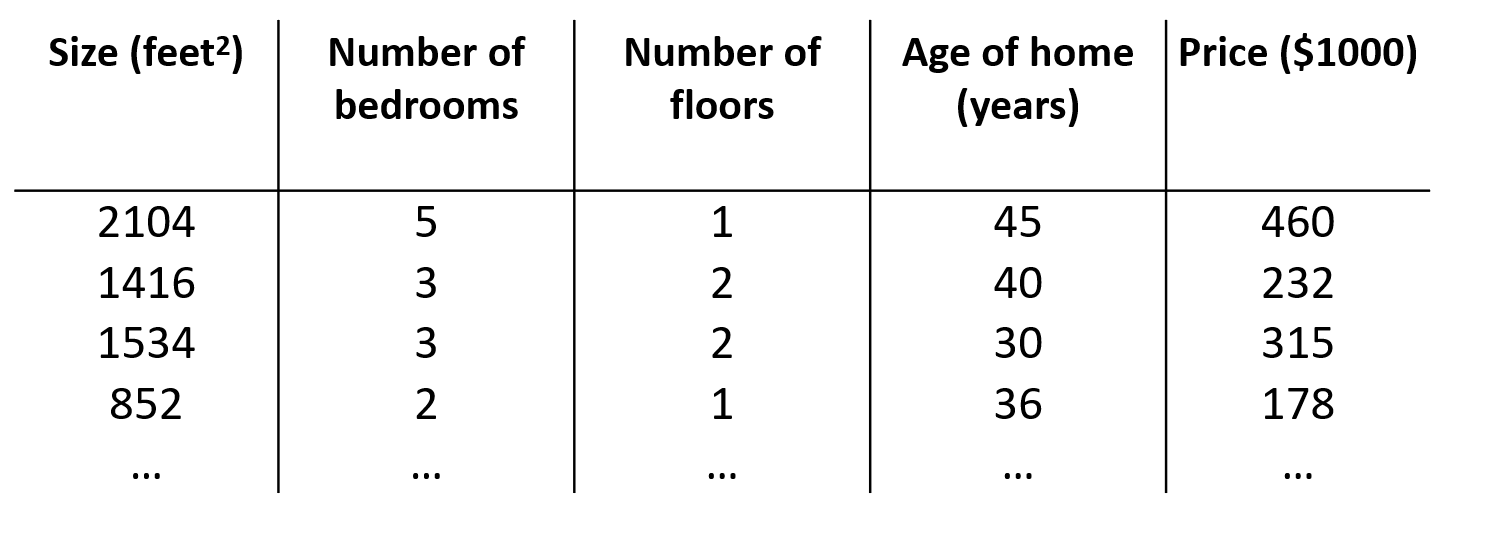在实际生活中，有卧室的数量，楼层的数量…都会影响房价的

符号约定：

n : 特征的数量
𝑥(𝑖) : 第 𝑖 个训练实例，是特征矩阵中的第𝑖行，是一个向量（ vector）
x j ( i ) : 特 征 矩 阵 中 第 𝑖 行 的 第 𝑗 个 , 第 𝑖 个 训 练 实 例 的 第 𝑗 个 特 征 x_j^{(i)} :特征矩阵中第 𝑖 行的第 𝑗个,第 𝑖 个训练实例的第 𝑗 个特征
支持多变量的假设函数h： ℎ𝜃(𝑥) = 𝜃0 + 𝜃1𝑥1 + 𝜃2𝑥2+. . . +𝜃𝑛𝑥𝑛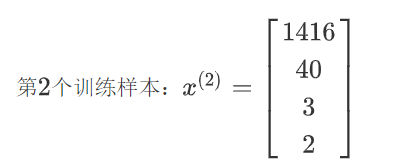第 二 个 样 本 的 第 二 个 特 征 和 第 三 个 特 征 ： 𝑥 2 ( 2 ) = 3 , 𝑥 3 ( 2 ) = 2 第二个样本的第二个特征和第三个特征：𝑥_2^{(2)} = 3, 𝑥_3^{(2)} = 2

假设函数中有𝑛 + 1个参数和𝑛个变量，为了使得公式能够简化，引入𝑥0 = 1，则公式为： ℎ𝜃(𝑥) = 𝜃0𝑥0 + 𝜃1𝑥1 + 𝜃2𝑥2+. . . +𝜃𝑛𝑥𝑛

此时模型中的参数是一个𝑛 + 1维的向量，任何一个训练实例也都是𝑛 + 1维的向量，特征矩阵𝑋的维度是 𝑚 ∗ (𝑛 + 1)。 所以公式可以简化为：ℎ𝜃(𝑥) = 𝜃^𝑇𝑋，其中上标𝑇代表矩阵转置

# 二、多变量梯度下降

与单变量线性回归一样的，在多变量线性回归中，构建一个代价函数，则代价函数是所有建模误差的平方和，以下是假设函数和代价函数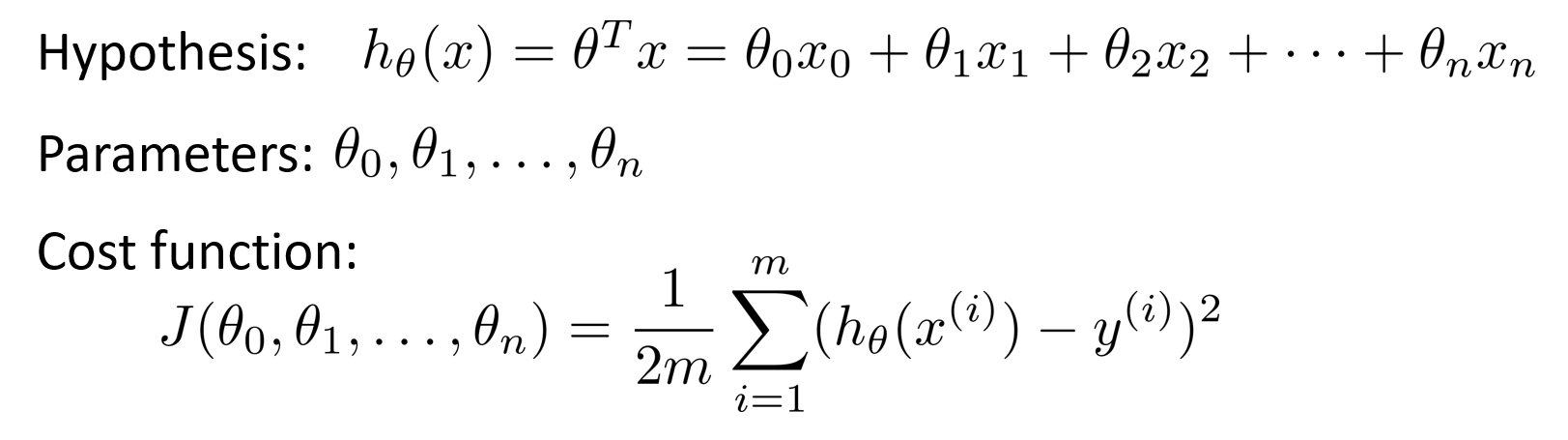在多变量线性回归中，目标和单变量线性回归问题中一样，是要找出使得代价函数最小的一系列参数

多变量线性回归的批量梯度下降算法为: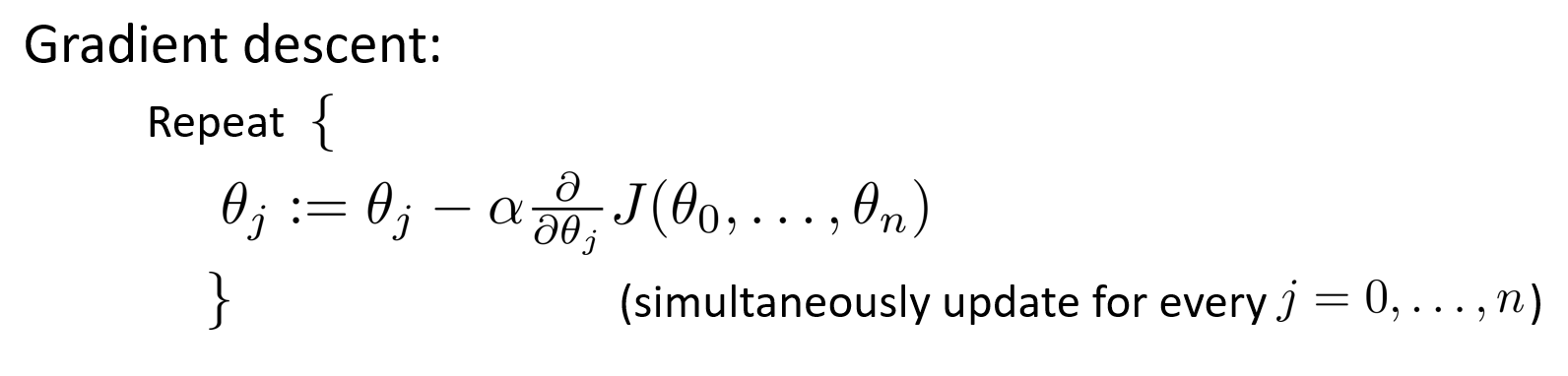单变量线性回归与多变量线性回归对比：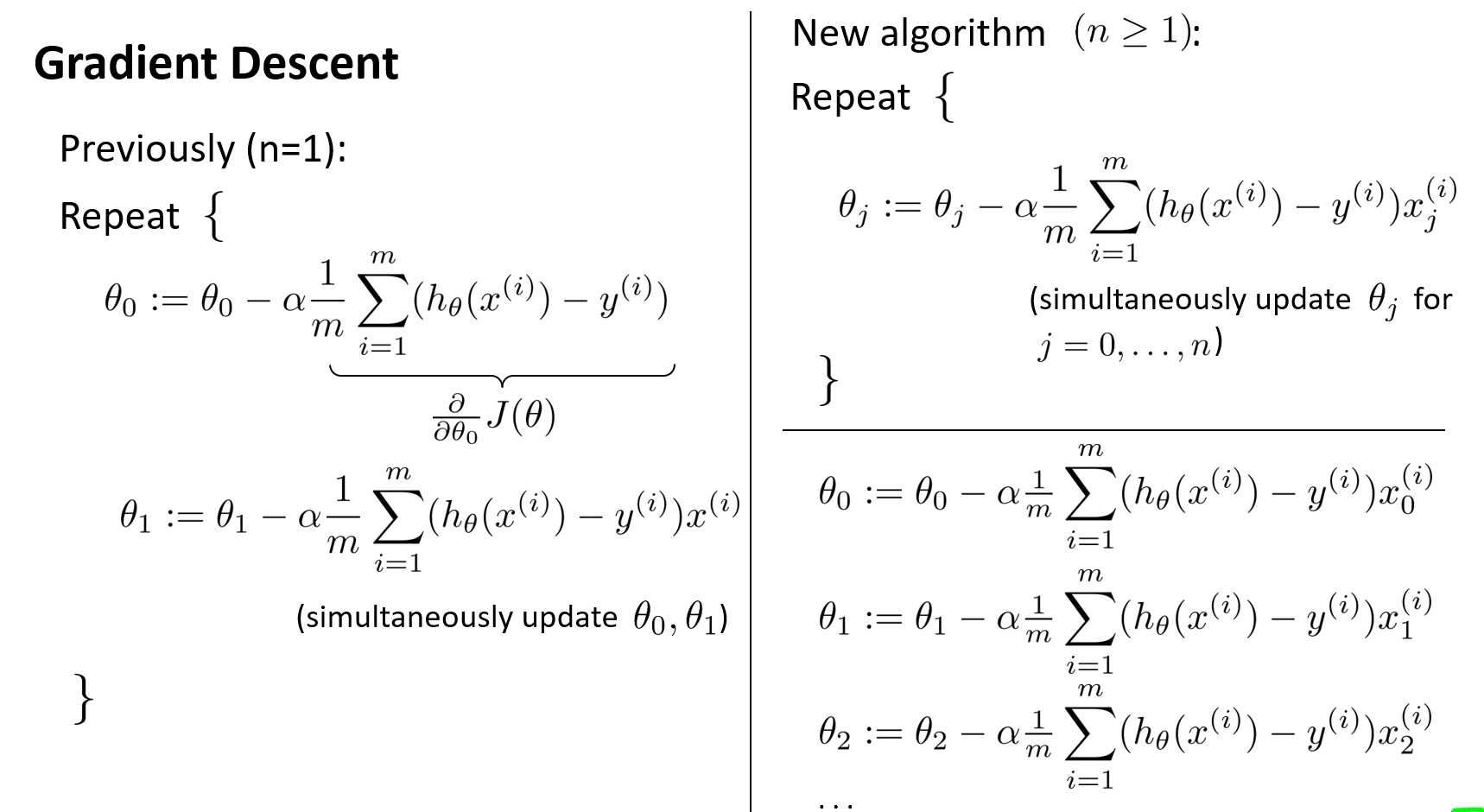开始随机选择一系列的参数值，计算所有的预测结果后，再给所有的参数一个新的值，如此循环直到收敛

代码

def computeCost(X, y, theta):
inner = np.power(((X * theta.T) - y), 2)
return np.sum(inner) / (2 * len(X))


## 1.特征缩放

对于处理多维特征问题，若保证了这些特征都具有相近的尺度，这将帮助梯度下降算法更快地收敛

eg:
假设有两个特征，房屋的尺寸和房间的数量，尺寸的值为 0-2000 平方英尺，房间数量的值则是 0-5，以两个参数分别为横纵坐标，绘制代价函数的等高线图，如图，图像显得很扁，梯度下降算法需要迭代多次才能收敛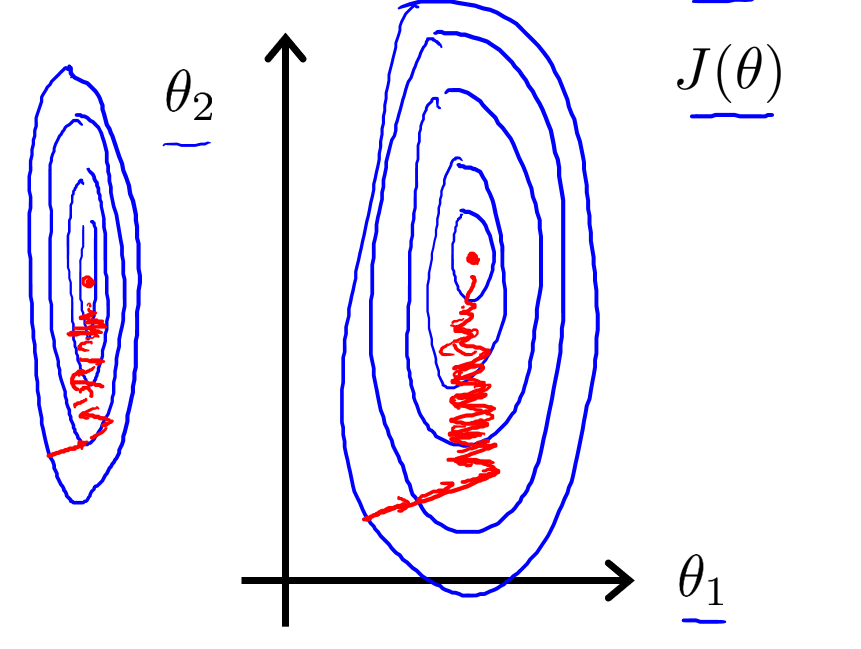特征缩放：将所有特征的尺度都尽量缩放到-1 到 1 之间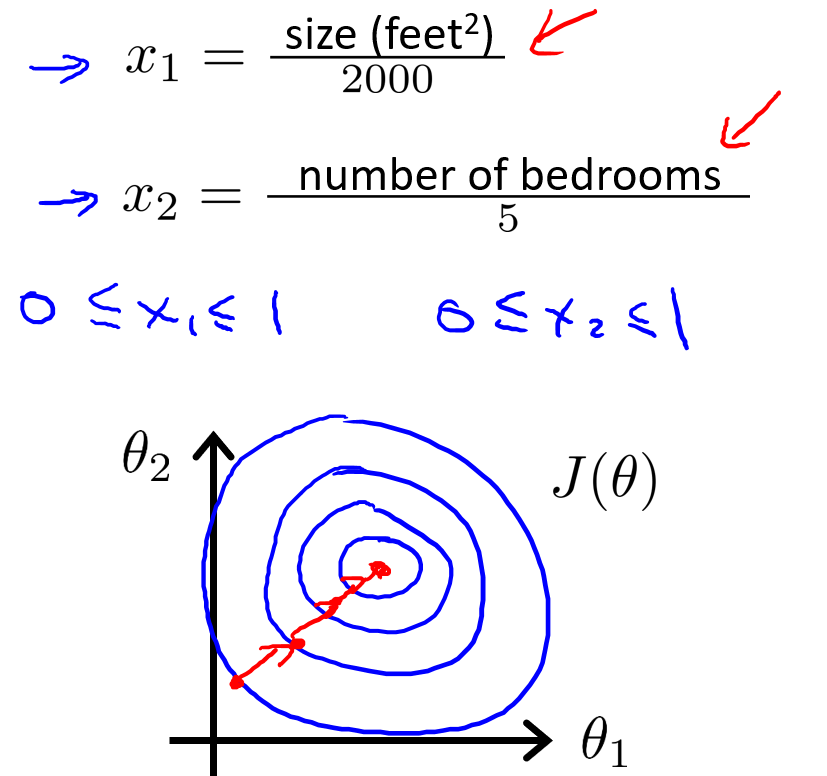在概率论中，归一化：𝑥𝑛 = (𝑥𝑛−𝜇𝑛)/ 𝑠𝑛，其中 𝜇𝑛是平均值， 𝑠𝑛是标准差

## 2.学习率

梯度下降算法收敛所需要的迭代次数根据模型的不同而不同，不能提前预知，可以绘制迭代次数和代价函数的图表来观测算法在何时趋于收敛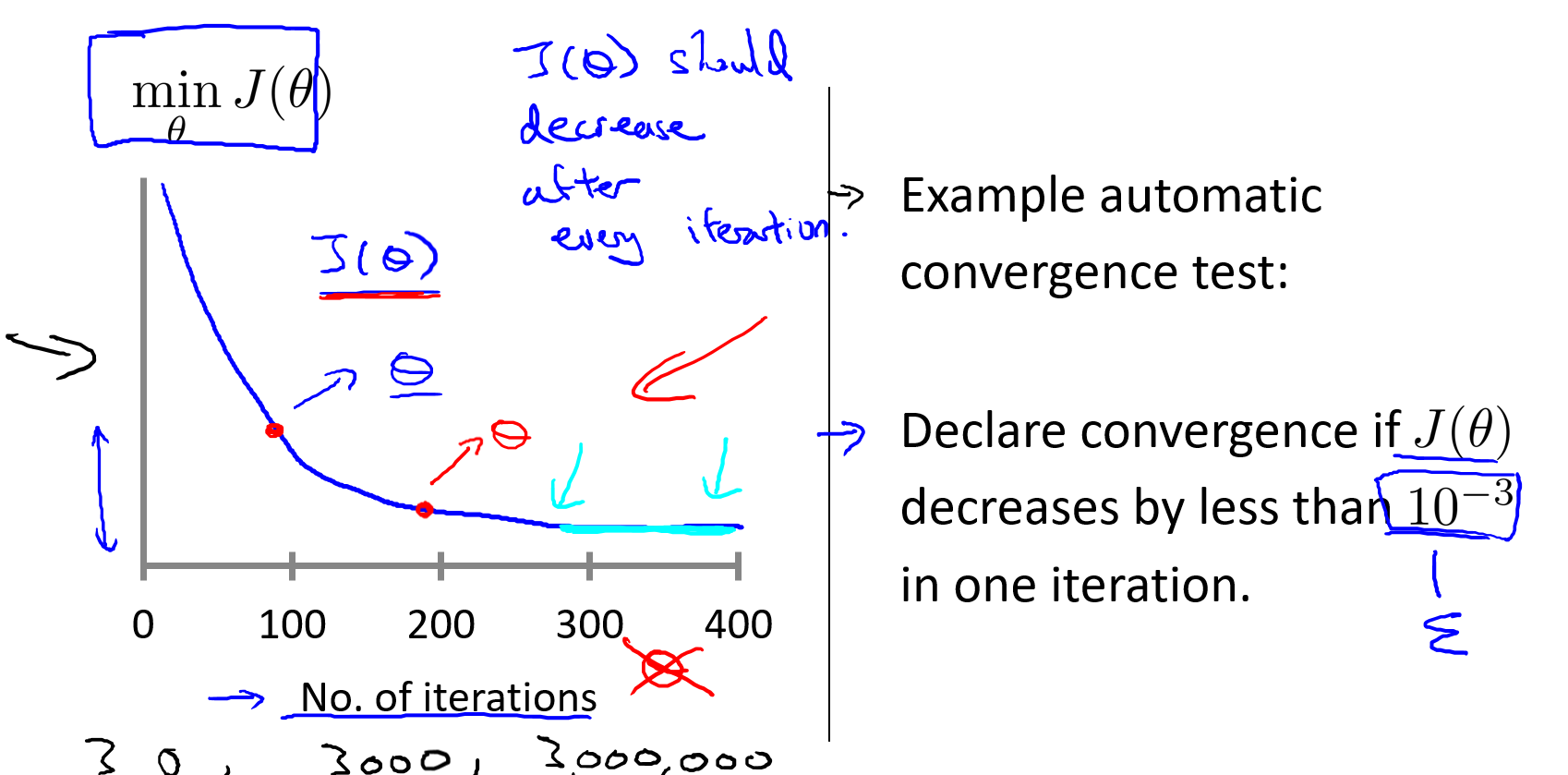也可以通过自动测试是否收敛的方法，例如将代价函数的变化值与某个阀值（例如 0.001）进行比较

一般推荐使用第一种画代价函数的图像，更直观，效果更好

前面我们知道梯度下降算法的每次迭代受到学习率的影响，如果学习率𝑎过小，则达到收敛所需的迭代次数会非常高；如果学习率𝑎过大，每次迭代可能不会减小代价函数，可能会越过局部最小值导致无法收敛，通常可以考虑尝试些学习率：𝛼 = 0.01， 0.03， 0.1， 0.3， 1， 3， 10 缓慢增加

# 三、多项式回归

## 1.特征选择

通过定义新的特征，可能会得到一个更好地模型
eg: 假设两个特征，分别是房子临街的宽度(frontage)和垂直宽度(depth)，则可以建立一个线性回归模型: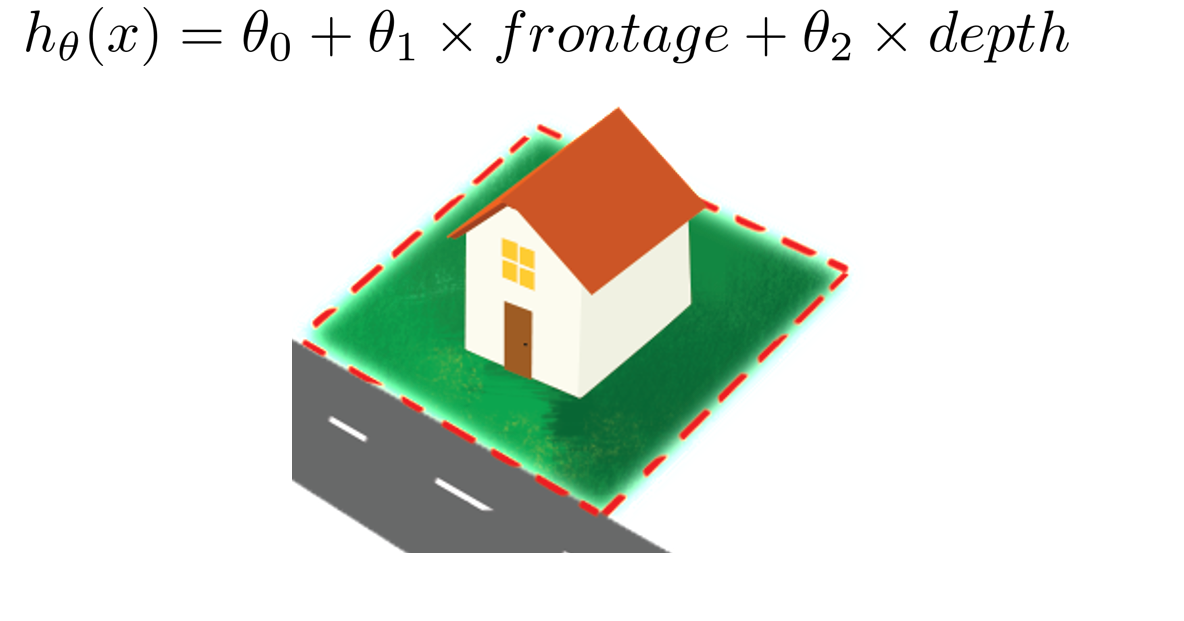但是当在运用线性回归时，不一定非要使用直接给出的x1 , x2，可以定义一个新的特征，例如在上述预测房价的例子中，可以认为能够决定房子大小的是所拥有的土地面积的大小，因此定义一个新特征area=𝑓𝑟𝑜𝑛𝑡𝑎𝑔𝑒 ∗ 𝑑𝑒𝑝𝑡ℎ, 则假设函数为：ℎ𝜃(𝑥) = 𝜃0 + 𝜃1𝑥

线性回归并不适用于所有数据，有时需要曲线来适应数据集，比如下图的数据集，如果用直线来拟合效果会很差的。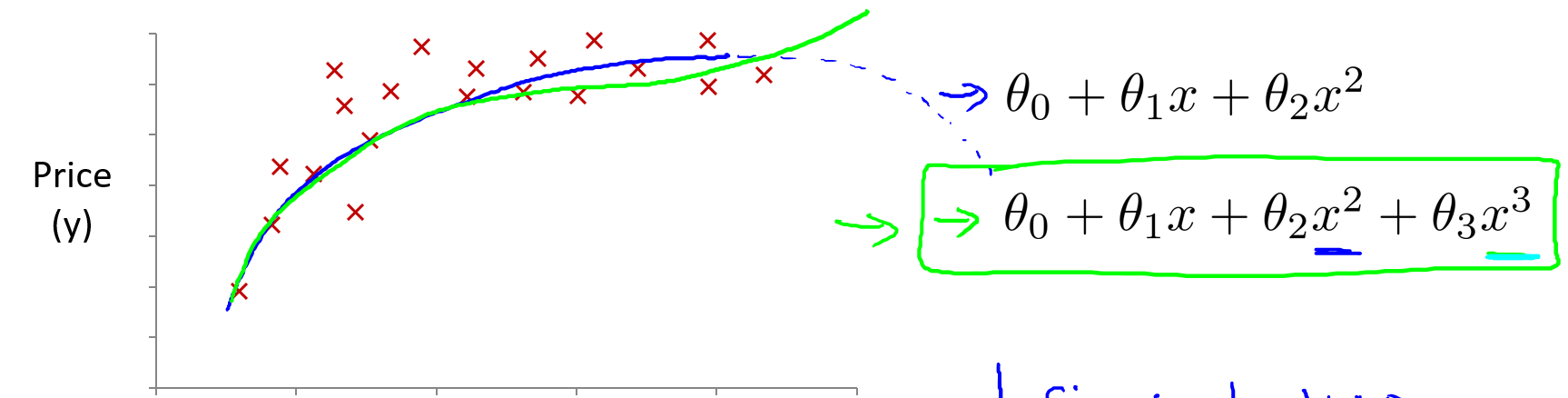在上图中二次函数对数据前半段拟合的很好，但是后半段由于二次函数的性质，曲线下降，不能很好地拟合后半段数据，所以这里三次或者多元函数更适合。可以使用多元线性回归(multiple linear regression)的方法将模型与数据进行拟合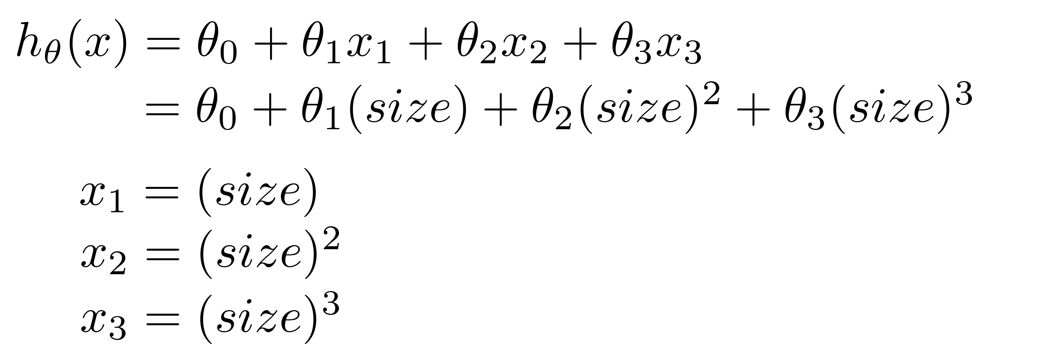另外，可以令：𝑥^2 = 𝑥2, 𝑥^3 = 𝑥3，从而将模型转化为线性回归模型

## 2.正规方程

前面，都是使用梯度下降算法，去解决代价函数最小化问题，但是对于某些线性回归问题，使用正规方程(只需要一步就能得到最优值)可以更加简便。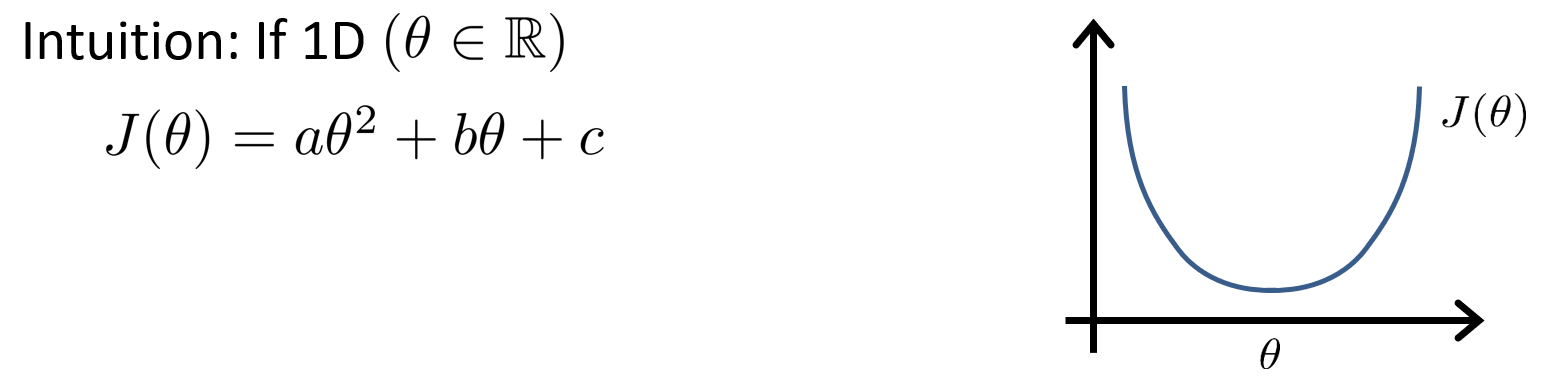正规方程是通过求解导数值为0的方程来找出使得代价函数最小的参数的，但是，参数𝜃一般不是一个实数，而是一个n+1维的向量，代价函数是这个向量的函数，将它的导数全部置0，求出全部的𝜃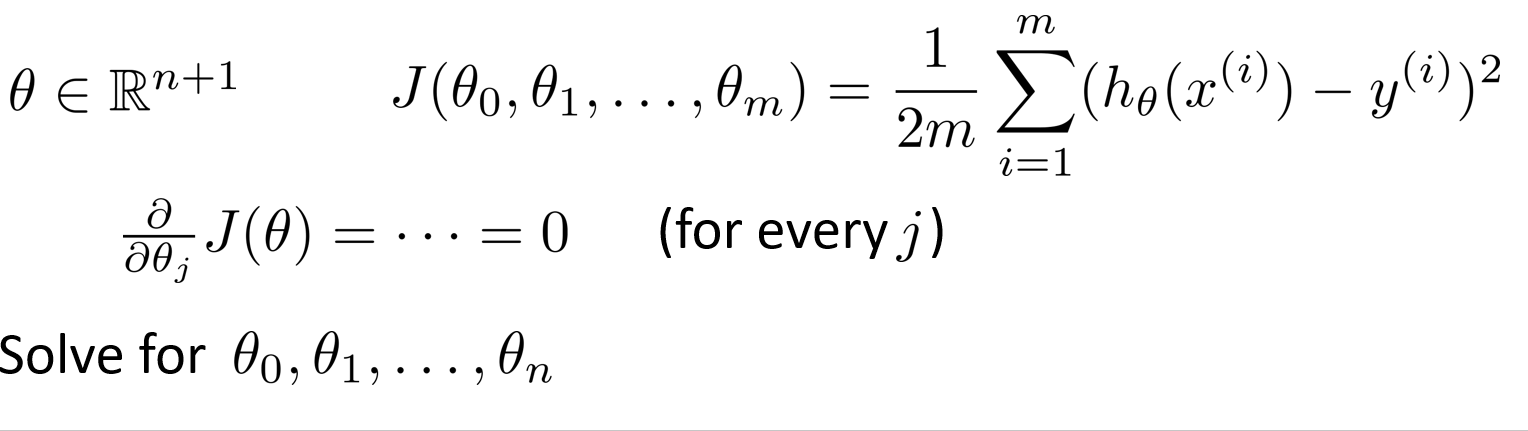假设训练集特征矩阵为 𝑋（包含了 𝑥0 = 1）并且训练集结果为向量 𝑦，则利用正规方程解出向量 𝜃 = (𝑋𝑇 𝑋)(−1)𝑋𝑇𝑦

注：上标 T 代表矩阵转置，上标-1 代表矩阵的逆

eg:
数据集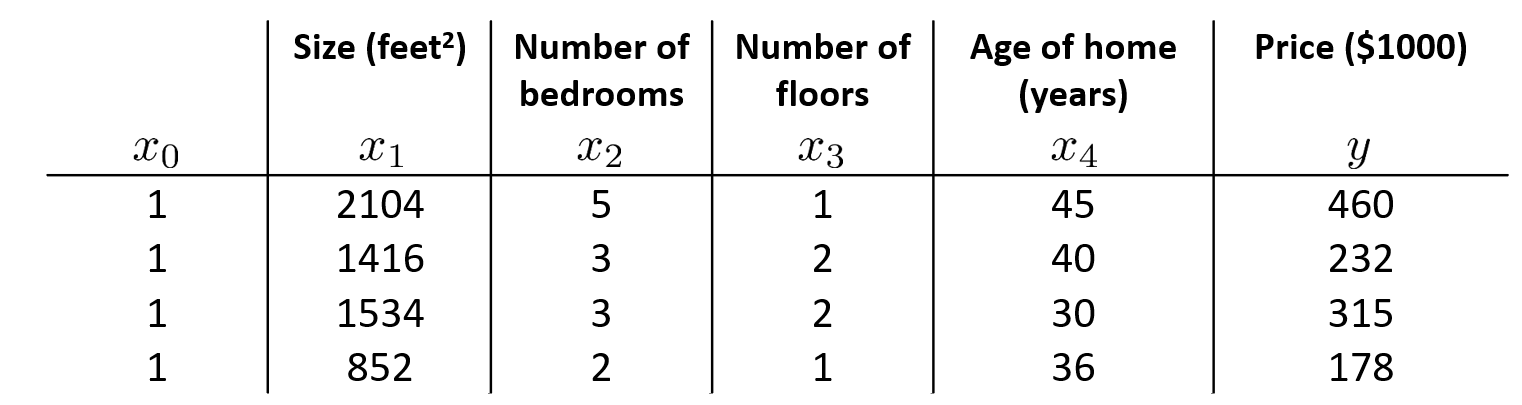了在上述数据中实现正规方程法，要在数据集中加上一列，以对应额外的特征变量x0
构建矩阵X ,它包含了训练样本的所有特征变量，以及向量y, 它包含了所有的预测值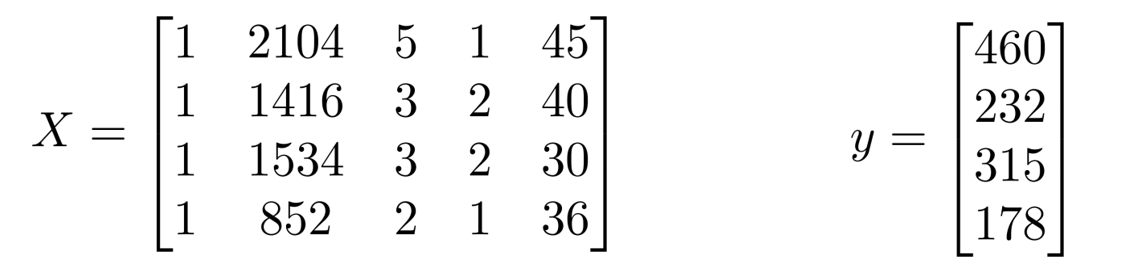则矩阵X和向量y通过下面的公式来计算使得代价函数最小化的θ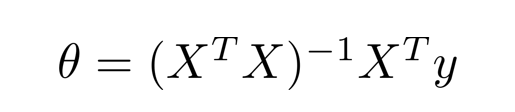即：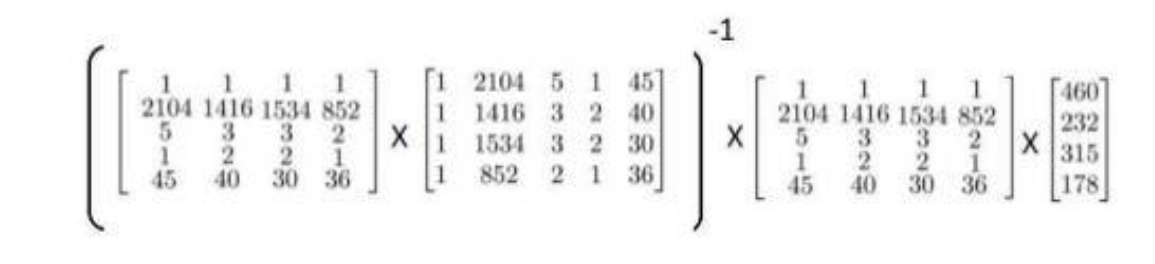矩阵X称为设计矩阵(design matrix)，构建设计矩阵的方法，就是取第一个训练样本，也就是第一个向量，取它的转置，作为设计矩阵的第一行，以此类推
注：要在数据集中加上一列，以对应额外的特征变量x0

梯度下降与正规方程的比较：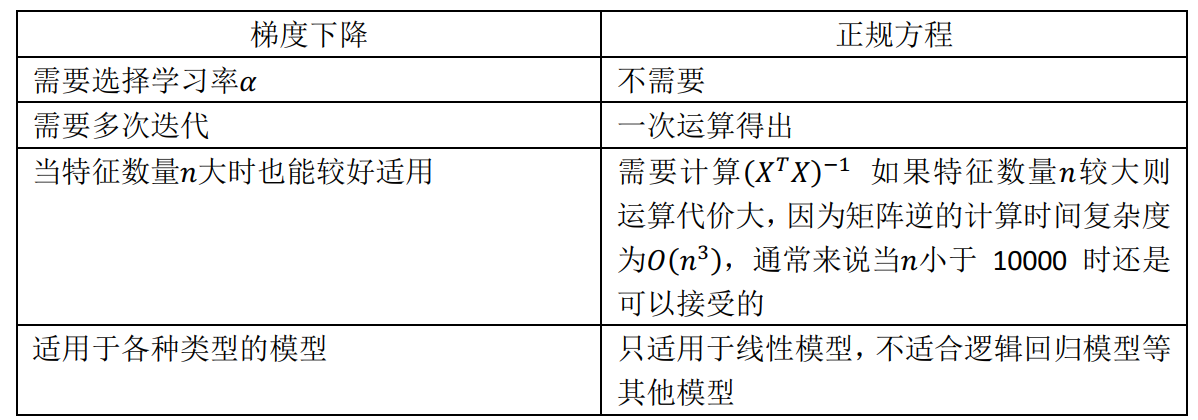只要特征变量的数目并不大，标准方程是一个很好的计算参数𝜃的替代方法。具体地说，只要特征变量数量小于一万，通常使用标准方程法，而不使用梯度下降法

正规方程的 python 实现：

import numpy as np
def normalEqn(X, y):
theta = np.linalg.inv(X.T@X)@X.T@y   # X.T@X 等价于 X.T.dot(X)
return theta


## 3.正规方程不可逆性

求解正规方程中参数θ的公式中，需要求矩阵(XT X )可逆，但是，如果不可能用这种方法求解，程序还是会解出正确的解

矩阵不可逆通常有两种最常见的原因

(1)特征值相关联，即𝑥1 = 𝑥2 ∗k
(2)特征值过多(在样本𝑚小于或等于特征值 n 的时候)

eg:
在预测住房价格时，如果𝑥1是以英尺为尺寸规格计算的房子， 𝑥2是以平方米为尺寸规格计算的房子(1 米等于3.28 英尺),这两个特征值将始终满足约束： 𝑥1 = 𝑥2 ∗(3.28)2,可以用一个线性方程，来描述两个相关联的特征值，矩阵𝑋′𝑋将是不可逆的

eg:
有𝑚等于 10 个的训练样本和有𝑛等于 100 的特征数量，要找到适合的(𝑛 + 1) 维参数矢量𝜃，尝试从 10 个训练样本中找到满足 101 个参数的值，花费的代价大

使用小数据样本以得到101 个参数，通常会使用一种叫做正则化的线性代数方法，通过删除某些特征或者是使用某些技术，来解决当𝑚比𝑛
小的时候的问题

当发现的矩阵𝑋′𝑋的结果是奇异矩阵，或者找到的其它矩阵是不可逆的首先应该通过观察所有特征检查是否有多余的特征(𝑥1和𝑥2是线性相关的，互为线性函数)，如果有多余的就删除掉，直到他们不再是多余的为止，如果特征数量实在太多，删除一些用较少的特征来反映尽可能多内容， 否则会考虑使用正规化方法

## 4.推导证明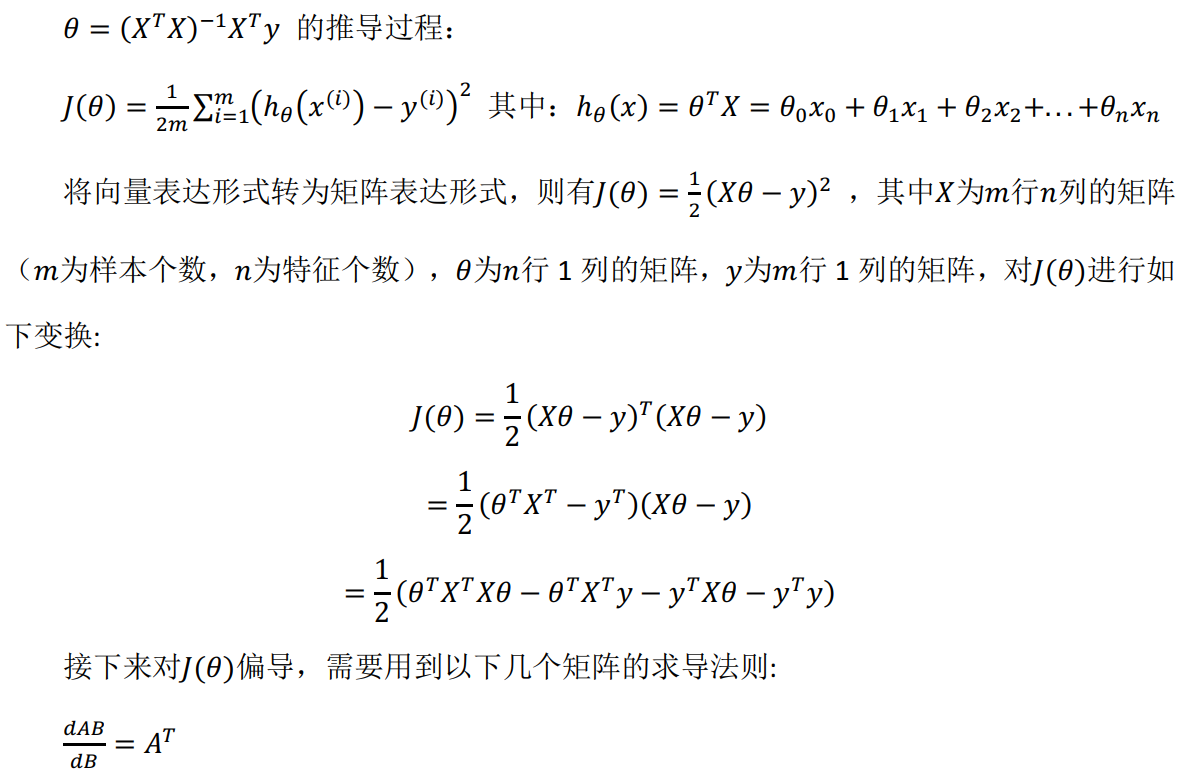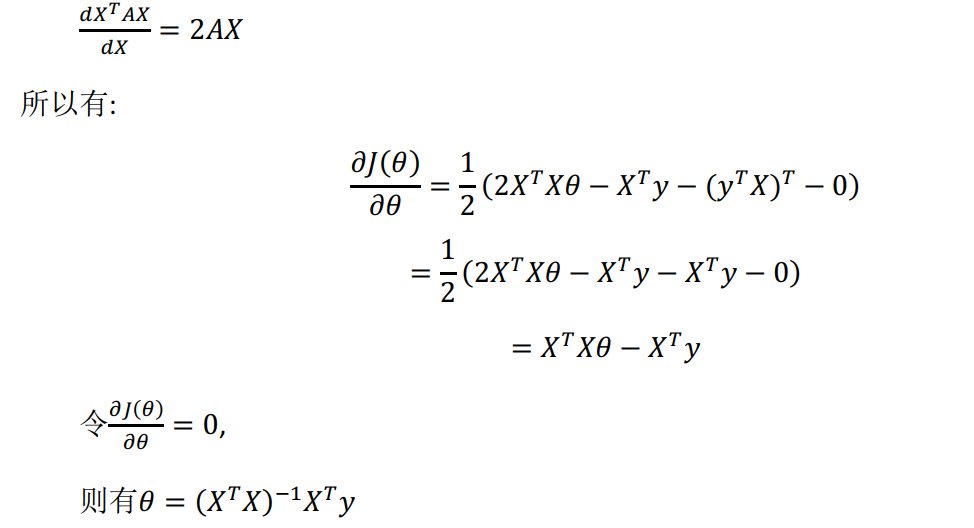# 总结

提示：这里对文章进行总结：

展开全文机器学习 Linux C/C++ python
• 一元线性回归线性回归多元线性回归多个因变量个自变量的回归如何从数据推断回归模型基本假设的合理性基本假设不成立时如何对数据进行修正回归诊断判断回归...
• Matlab一元非线性回归分析的分析步骤与一元线性回归分析的步骤类似：大体分为以下几步：(1)绘制x,y的散点图，分析散点图的走势；(2)根据散点图的走势，确定回归方程的具体形式，特别是参数个数的设定和设定初始值；...

Matlab一元非线性回归分析的分析步骤与一元线性回归分析的步骤类似：

大体分为以下几步：

(1)绘制x,y的散点图，分析散点图的走势；

(2)根据散点图的走势，确定回归方程的具体形式，特别是参数个数的设定和设定初始值；

(3)调用NonLinearModel的fit方法进行模型拟合；

(4)模型改进，去除异常值的操作；

(5)进行残差分析，验证模型。

下面以某商品的数量与定价为例，进行实例展示；

(1)绘制x,y的散点图，分析散点图的走势；

x=data(:,2);%提取列数据自变量数据

y=data(:,3);%提取列数据因变量数据

figure;

plot(x,y,'ko');%绘制散点图

xlabel('数量');

ylabel('价格');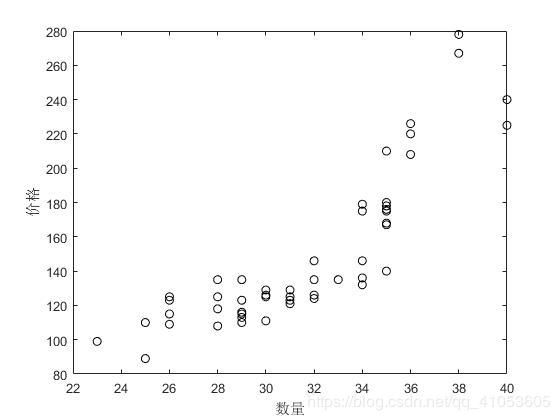图1 散点图

(2)根据散点图的走势，确定回归方程的具体形式，特别是参数个数的设定和设定初始值；

%建立一元非线性回归方程

% yi=f(xi;b1,b2)+ai

% ai~N(0,aa^2),i=1,2,..n

price=@(beta,x)beta(1)./(1-beta(2)*x);%根据散点图趋势建立方程f(x)=b1./(1-b2*x),方程形式并不唯一。

beta0=[120,0.008];%beta0为b1,b2的初始值。根据x的取值范围，x在22与40之间，y>0，故需b2<0.25。

%根据y值是84到280的不等分布，主要集中在110到130，故b1取值120较合适，根据方程，b2取0.008即可。

opt=statset;%创建结构体变量类

opt.Robust='on';%开启回归稳健性方法

nlm1=NonLinearModel.fit(x,y,price,beta0,'Options',opt);

%y=41.459./(1-0.02213*x)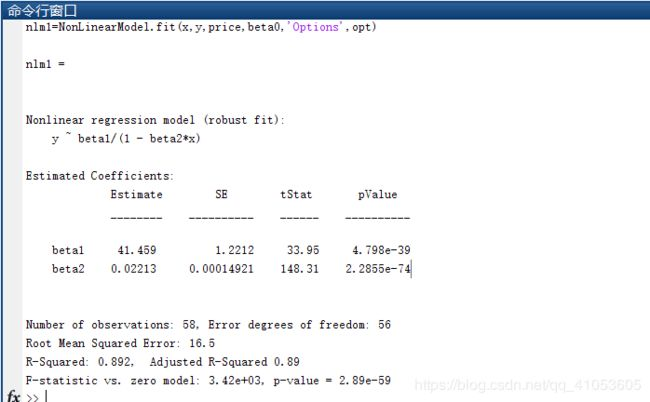(3)调用NonLinearModel的fit方法进行模型拟合；

xnew=linspace(20,40,50)';%取50个x的值在20到40之间

ynew=nlm1.predict(xnew);%进行ynew预测

figure;

plot(x,y,'ko');

hold on;

plot(xnew,ynew,'linewidth',2.5);

xlabel('数量');

ylabel('价格');

legend('原始数据散点','非线性回归曲线');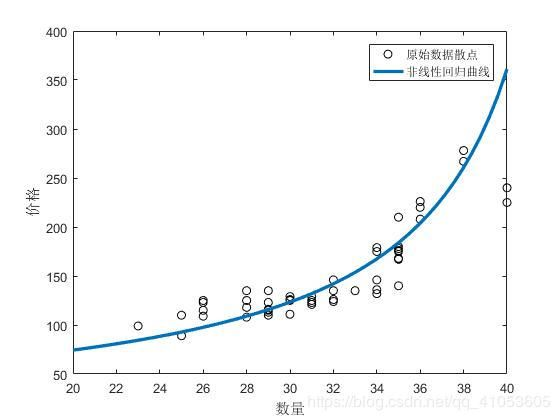图2 模型拟合效果

(4)模型改进，去除异常值的操作；

异常值的诊断。NonlinearModel类对象的Residuals属性值中有标准化残差和学生化残差值。这里通过学生化残差查询异常值

Res2=nlm1.Residuals;

Res_Stu2=Res2.Studentized;

id2=find(abs(Res_Stu2)>2);

%properties(nlm1);%可以查询nlm1的属性

%去除异常值重新构建回归模型

nlm2=NonLinearModel.fit(x,y,price,beta0,'Exclude',id2,'options',opt);

%y=41.394./(1-0.22195*x)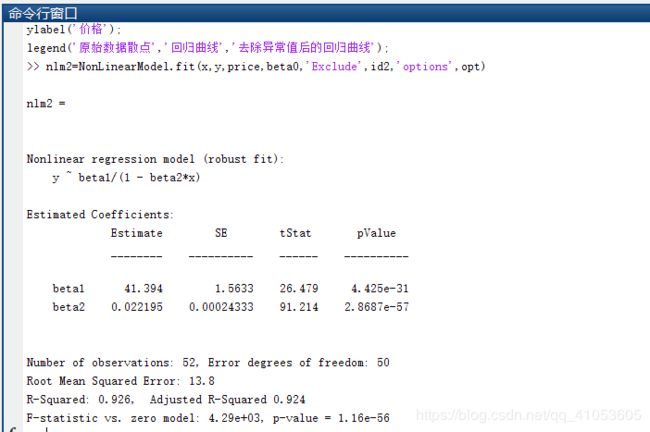xnew=linspace(20,40,50)';

y1=nlm1.predict(xnew);

y2=nlm2.predict(xnew);

figure;

plot(x,y,'ko');

hold on;

plot(xnew,y1,'r--','linewidth',2);

plot(xnew,y2,'b.','linewidth',2);

xlabel('数量');

ylabel('价格');

legend('原始数据散点','回归曲线','去除异常值后的回归曲线');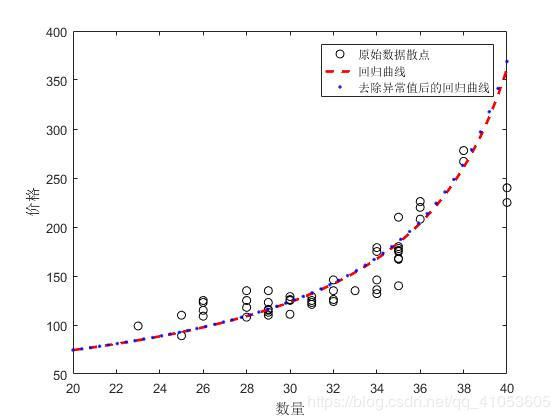图3 去除异常值与未去除异常值的拟合对比

(5)进行残差分析，验证模型。

调用的是NonLinearModel类中的plotResiduals方法。残差分析有比较多的方法，这里以残差直方图和残差正态概率图为例。

%回归诊断

figure;

subplot(1,2,1);

nlm1.plotResiduals('histogram');

title('(a)残差直方图');

xlabel('残差r');

ylabel('f(r)');

subplot(1,2,2);

nlm1.plotResiduals('probability');

title('(b)残差正态概率图');

xlabel('残差');

ylabel('概率');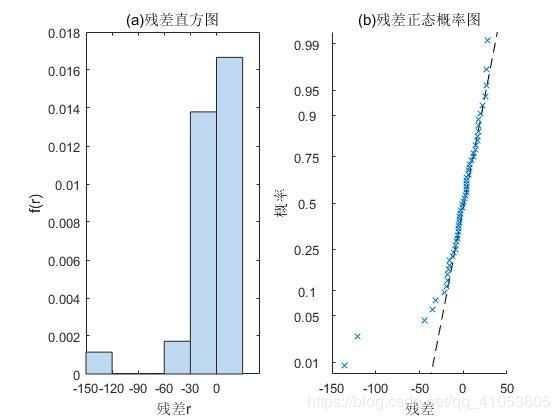图4 残差直方图和残差正态概率图

原始数据

order

数量

价格

1

25

89

2

28

108

3

26

115

4

23

99

5

26

109

6

32

124

7

35

210

8

30

126

9

34

132

10

29

110

11

33

135

12

36

226

13

34

136

14

36

220

15

36

208

16

30

125

17

32

146

18

30

129

19

35

140

20

29

116

21

31

123

22

32

126

23

28

135

24

36

208

25

40

240

26

28

125

27

29

113

28

36

208

29

32

135

30

38

267

31

40

225

32

38

278

33

28

118

34

32

124

35

34

146

36

36

208

37

30

111

38

29

115

39

36

208

40

29

123

41

25

110

42

29

135

43

26

123

44

23

99

45

26

125

36

35

180

37

35

178

38

35

175

39

35

176

40

35

180

41

35

178

42

34

175

43

35

168

44

35

167

45

34

179

43

31

129

44

31

121

45

31

125

展开全文• 常用的非线性分析方法由3终，包括非参数回归分析、转换变量回归分析以及非线性回归分析。 12.1非参数回归分析 非参数回归分析与前面讲述的回归方式区别很大，是一种探索性工具，通常不会像其他回归方法一样形成一...
• 紧接着，我们介绍多项式回归分析（polynomial regression问题），一种具有非线性关系的多元线性回归问题。最后，我们介绍如果训练模型获取目标函数最小化的参数值。在研究一个大数据集问题之前，我们先从一个小问题...
• 在回归分析中，只包括一个自变量和一个因变量，且二者的关系可用一条曲线近似表示，则称为一元非线性回归分析。 一元二次方程： 一元三次方程： 一元 n 次方程： （二）案例 1.建模逻辑 一元非线性回归方程转为...
• 多变量线性相关分析 现实世界中的数据科学 (Data Science in the Real World) This article aims to present two ways of calculating non linear correlation between any number of discrete variables. The ...python java 机器学习 leetcode 数据结构
• 1、可线性化的非线性分析变量之间的非线性形式较，较为常见的形式如下图：非线性形式的变量关系一般可以通过变量代换或转换的方式转化为线性关系。横纵轴所代表变量之间的关系为幂函数形式的关系，即可建立的模型...
• r语言：fit1 = lm(y-x，data=xxx)，fit2 = lm(y-x+I(x^2)，data=xxx） summary(fit1) plot(fit1)\ 多元回归 相关关系：cor(xxx) 热图：heatmap(cor(xxx)) 非线性回归 1. 根据已知的数据绘制散点图，以此分析出数据的...
• ## 非线性回归分析及其Matlab实现

万次阅读 多人点赞 2020-02-23 15:14:22
回归分析简介 ...如果自变量与因变量之间的函数关系是线性函数，则称之为线性回归分析，否则称之为非线性回归分析。 线性回归的函数表达式一般表示为： 对于非线性回归分析，需要根据实际情况来确定函...
• matlab多元参数非线性回归模型代码输出高斯过程 输出回归 在输出回归（目标，多变量响应回归）中，我们旨在预测个实值输出变量。 一种简单的方法可能是使用单个输出回归模型的组合。 但是这种方法有...
• 我们之前介绍的线性回归是一个变量（特征）对预测价格的影响，像这个例子中是房子的大小对价格的影响 下面会介绍更为复杂的情况就是变量（特征）对于房子价格的影响 在这个多变量的图中，下面解释了每个符号...机器学习 人工智能
• 在利用SPSS进行非线性回归分析的操作过程中，在给定函数模型的条件下，需要给函数赋初值，按照教程(单击“参数”按键，弹出“非线性回归：参数”对话框，设置参数的开始值a=13，b=-6.5，c=-1.5)进行操作时，发现开始...
• 数据回归-响应变量存在缺失时非线性半参数回归模型的经验似然推断.pdf
• ## 多元线性回归分析

千次阅读 2021-10-21 09:24:41
回归分析中，如果有两个或两个以上的自变量，就称为多元回归。事实上，一种现象常常是与个因素相联系的，由个自变量的最优组合共同来预测或估计因变量，比只用一个自变量进行预测或估计更有效，更符合实际。...sklearn python
• sklearn实现非线性回归模型前言： sklearn实现非线性回归模型的本质是通过线性模型实现非线性模型，如何实现呢？sklearn就是先将非线性模型转换为线性模型，再利用线性模型的算法进行训练模型。一、线性模型解决非...
• 针对经典线性回归模型无法反映变量间的非线性关系,不适宜预测有模糊数的煤炭发热量的问题,提出了一种基于三角模糊数的多元非线性回归的煤炭发热量预测模型。以我国新疆伊犁地区煤炭工业分析为建模数据和模型检验数据...
• matlab多元非线性回归及显著性分析给各位高手：小弟有一些数据需要回归分析(非线性)及显著性检验(回归模型，次要项，误差及失拟项纯误差，F值和P值)，求大侠帮助，给出程序，不胜感激。模型：DA TA=... %DA TA前三列...
• 以实测数据为依据,采用IBM SPSS Statistics 24.0软件进行Langmuir方程参数线性回归与非线性回归的对比分析。结果表明:线性回归方法不满足相应曲线因变量的残差平方和最小,线性回归方法中对变量由无理数到有限小数的...
• 但是，在非线性回归中，每个参数的正确原假设值取决于预期函数以及参数在预期函数中的位置。 步骤 3：确定模型对数据的拟合优度 要确定模型对数据的拟合优度，请检查模型汇总表和失拟表中的统计量。 S 使用 S...
• 2017-08-02@erixhao 技术极客TechBooster ...AI 机器学习第二篇 - 非线形回归分析。我们上文深入本质了解了机器学习基础线性回归算法后，本文继续研究...非线性回归之后，我们会继续经典机器学习算法包括决策人工智能 AI 机器学习 算法
• ## SPSS（七）非线性回归过程

万次阅读 多人点赞 2019-05-17 18:56:44
SPSS（七）非线性回归过程 直线关系毕竟是较少数的情形，当因变量和自变量呈曲线关系，我们可以使用回归分析衍生方法----曲线拟合过程 但是使用曲线拟合过程还是存在局限性的 只能分析一个自变量 变量变换的局限...自定义损失函数 最小一乘法
• 起步非线性回归是线性回归的延伸，线性就是每个变量的指数都是 1，而非线性就是至少有一个变量的指数...化非线性回归为线性回归通过变量代换，可以将很非线性回归转化为线性回归。比如目标函数假设是 y = b0 + b...
• 1. 线性回归 1.1 导库 import numpy as np import keras from keras.models import Sequential from keras.layers import Dense import matplotlib.pyplot as plt 1.2 生成数据   其中np.random.rand是生成[0, 1]...Keras
• 之前的文章中都是给大家写的变量间线性关系的做法，包括回归和广义线性回归变量间的非线性关系其实是很常见的，今天给大家写写如何拟合论文中常见的非线性关系。包括多项式回归Polynomial regression和样条回归...r语言 R...